Math Calculators, Lessons and Formulas

It is time to solve your math problem

mathportal.org

# Triangle calculator

This calculator can compute area of the triangle, altitudes of a triangle, medians of a triangle, centroid, circumcenter and orthocenter.

Triangle in coordinate geometry
Input vertices and choose one of seven triangle characteristics to compute.
show help ↓↓ examples ↓↓
 Area (default) Medians Altitudes Centroid (intersection of medians) Incenter (center of the incircle) Circumcenter (center of circumscribed circle) Orthocenter (intersection of the altitudes)
Hide graph
Hide steps
Find approximate solution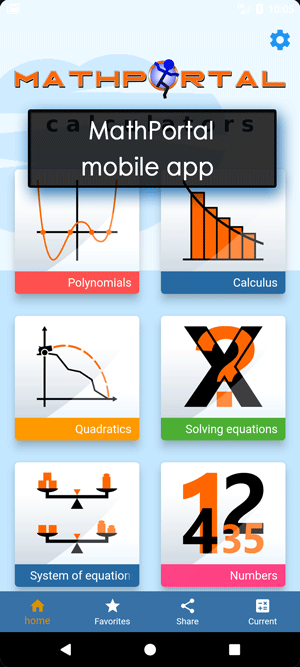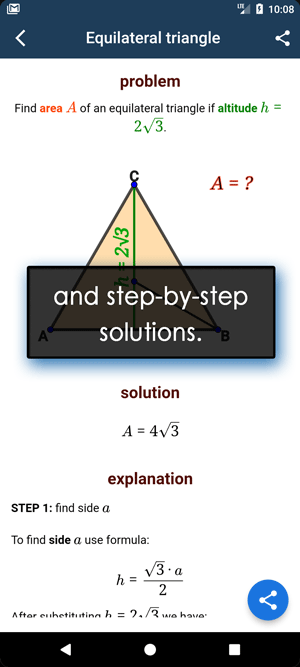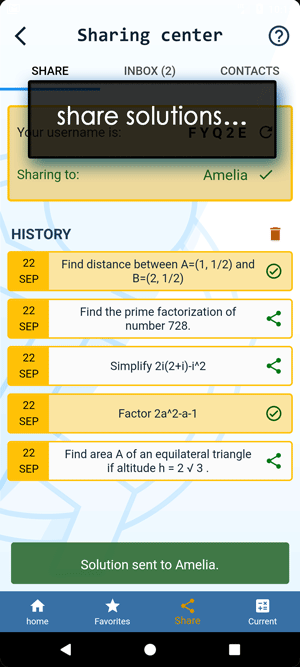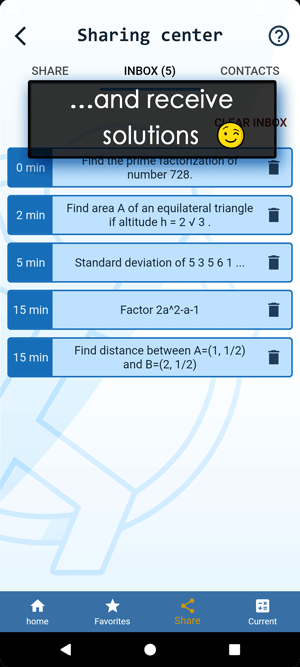working...
examples
example 1:ex 1:
Find area of a triangle whose vertices are $(4, 4), (-2, 3)$ and $(-4, -5)$.
example 2:ex 2:
Find area of a triangle whose vertices are $\left(\frac{4}{3}, 3.5\right), \left(8, -\frac{1}{2}\right)$ and $(-7, 5.2)$.
example 3:ex 3:
Find altitudes of a triangle whose vertices are $(1,1), (3,5)$ and $(-10, 8)$.
example 4:ex 4:
Find circumcenter of a triangle whose vertices are $(-2,-5), (3,4)$ and $(10, -3)$.

# Formulas and examples for triangle

## Area of the triangle?

The area of a triangle whose vertices are $A(x_A, y_A), B(x_B, y_B)$ and $C(x_C, y_C)$ is given by :

$${\color{blue}{ K = \frac12|x_A(y_B - y_C) + x_B(y_C - y_A) + x_C(y_A-y_B)| }}$$

Example:

Find the area of the triangle whose vertices are $A(2, 4), B(3, -1)$ and $C(-3, 3)$.

Solution:

In this example we have: $x_A = 2,~~ y_A = 4,~~ x_B = 3,~~ y_B = -1, x_C = -3,~~ y_C = 3$. So we have: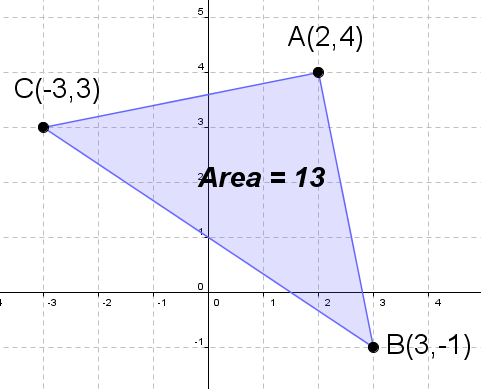\begin{aligned} K & = \frac12|x_A(y_B - y_C) + x_B(y_C - y_A) + x_C(y_A-y_B)| \\ K & = \frac12|2(-1 - 3) + 3(3 - 4) -3(4-(-1))| \\ K & = \frac12|2\cdot(-4) + 3\cdot(-1) -3\cdot5| \\ K & = \frac12|-8 - 3 -15| \\ K & = \frac12|-26| \\ K & = \frac12\cdot26 \\ K & = 13 \end{aligned}

## Centroid of the triangle?

The centroid of a triangle whose vertices are $A(x_A, y_A), B(x_B, y_B)$ and $C(x_C, y_C)$ is given by :

$${\color{blue}{ (x,y) = \left(\frac{x_A + x_B + x_C}{3}, \frac{y_A + y_B + y_C}{3}\right) }}$$

Example:

Find the centroid of the triangle whose vertices are $A(2, 4), B(3, -1)$ and $C(-3, 3)$.

Solution: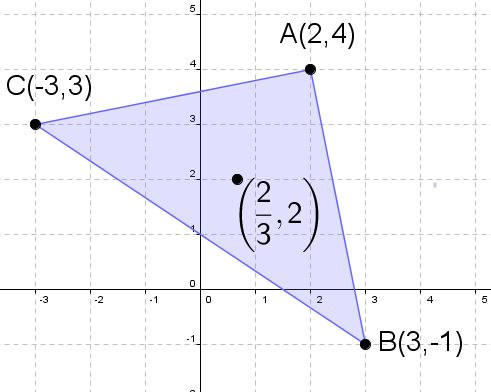Using the same $x_A, y_A, x_B, y_B, x_C, y_C$, as in previous example we have:

\begin{aligned} (x,y) & = \left(\frac{x_A + x_B + x_C}{3}, \frac{y_A + y_B + y_C}{3}\right) \\ (x,y) & = \left(\frac{2 + 3 - 3}{3}, \frac{4 - 1 + 3}{3}\right) \\ (x,y) & = \left(\frac{2}{3}, 2\right) \\ \end{aligned}
Search our database of more than 200 calculators